• 数字签名代码
千次阅读
2021-06-21 19:06:36

目录

数字签名概述

数字签名和手写签名的不同

数字签名与消息加密/认证

数字签名的步骤

Go中使用Rsa进行数字签名

crypto/rsa包

crypto/sha512包

代码

截图

参考

# 数字签名概述

手写签名是一种传统的确认方式，如写信、签订协议、支付确认等。在数字系统中同样有签名应用的需求，如假定A发送一个认证的信息给B，如果没有签名确认的措施，B可能伪造一个不同的消息，但声称是从A收到的；或者为了某种目的，A也可能否认发送过该消息。很显然，数字系统的特点决定了不可能沿用原先的手写签名方法来实现防伪造或抵赖，这就是提出了如何实现数字签名的问题。

数字签名（Digital Signature是电子信息技术发展的产物，是针对电子信息 的一种签名确认方法，它是利用数学方法和密码算法对该电子信息进行关键信息提取并进行加密而形成的，所要达到的目的是：对数字对象的合法化、真实性进行标记，并提供签名者的承诺。

数字化信息的认证性、完整性和不可否认性是实现信息化的基本要求，也决定信息化的普及和推广。数字签名是满足上述要求的主要手段之一也是现代密码学的主要研究内容之一。

数字签名基于两条基本的假设：

一是私钥是安全的，只有其拥有者才能获得；

二是产生数字签名的惟一途径是使用私钥

## 数字签名和手写签名的不同

• 签名手写签名是被签文件的物理组成部分数字签名是连接到被签消息上的数字串
• 传输方式数字签名和所签名的消息能够在通信网络中传输手写签名使用传统的安全方式传输。
• 验证手写签名是通过将它与真实的签名进行比较来验证；而数字签名是利用已经公开的验证算法来验证
• 数字签名的复制是有效的；而手写签名的复制品是无效的！！！
• 手书签名是模拟的，且因人而异数字签名是0和1的数字串，因消息而异

## 数字签名与消息加密/认证

与消息加密不同：消息加密和解密可能是一次性的，它要求在解密之前是安全的；而一个签字的消息可能作为一个法律上的文件，如合同等，很可能在对消息签署多年之后才验证其签字，且可能需要多次验证此签字。
与消息认证不同数字签名也是一种消息认证技术，它属于非对称密码体制，消息认证码属于对称密码体制，所以消息认证码的处理速度比数字签名快得多。但是，消息认证码无法实现不可否认性。

# 数字签名的步骤

1. 系统初始化生成数字签名方案用到的所有参数（公私钥对）。
2. 签名生成算法用户利用给定的算法对消息产生签名。
3. 签名验证算法验证者利用公开的验证方法对给定消息的签名进行验证，得出签名的有效性。

数字签名的算法有RSA、ECC等。本文介绍RSA数字签名的实现，第一步的公私钥对生成见RSA加密解密详解与代码

# Go中使用Rsa进行数字签名

## crypto/rsa包

func SignPKCS1v15(rand io.Reader, priv *PrivateKey, hash crypto.Hash, hashed []byte) (s []byte, err error)

SignPKCS1v15使用RSA PKCS#1 v1.5规定的RSASSA-PKCS1-V1_5-SIGN签名方案计算签名。注意hashed必须是使用提供给本函数的hash参数对（要签名的）原始数据进行hash的结果。

func VerifyPKCS1v15(pub *PublicKey, hash crypto.Hash, hashed []byte, sig []byte) (err error)

VerifyPKCS1v15认证RSA PKCS#1 v1.5签名。hashed是使用提供的hash参数对（要签名的）原始数据进行hash的结果。合法的签名会返回nil，否则表示签名不合法。

## crypto/sha512包

func Sum512(data []byte) [Size]byte

返回数据的SHA512校验和。

## 代码

签名

1. 获取私钥
2. 使用x509.ParsePKCS1PrivateKey解析
3. 使用sha512.Sum512获取明文校验和
4. 使用rsa.SignPKCS1v15进行签名
func RsaSign(plainText []byte, priFilePath string) ([]byte,error) {
// get pem.Block
block,err := util.GetKey(priFilePath)
if err != nil{
_, file, line, _ := runtime.Caller(0)
return nil,util.Error(file,line+1,err.Error())
}
priKey,err := x509.ParsePKCS1PrivateKey(block.Bytes)
if err != nil{
_, file, line, _ := runtime.Caller(0)
return nil,util.Error(file,line+1,err.Error())
}
// calculate hash value
hashText := sha512.Sum512(plainText)
// Sign with hashText
signText, err := rsa.SignPKCS1v15(rand.Reader, priKey, crypto.SHA512, hashText[:])
if err != nil{
_, file, line, _ := runtime.Caller(0)
return nil,util.Error(file,line+1,err.Error())
}
return signText,nil
}

验证

1. 获取公钥
2. 使用x509.ParsePKIXPublicKey解析
3. 使用sha512.Sum512获取明文校验和
4. 使用rsa.VerifyPKCS1v15进行验证
func RsaVerify(plainText []byte, pubFilePath string,signText []byte) error {
// get pem.Block
block,err := util.GetKey(pubFilePath)
if err != nil{
_, file, line, _ := runtime.Caller(0)
return util.Error(file,line+1,err.Error())
}
// x509
pubInter,err := x509.ParsePKIXPublicKey(block.Bytes)
if err != nil{
_, file, line, _ := runtime.Caller(0)
return util.Error(file,line+1,err.Error())
}
pubKey := pubInter.(*rsa.PublicKey)
// hashText to verify
hashText := sha512.Sum512(plainText)
err = rsa.VerifyPKCS1v15(pubKey,crypto.SHA512,hashText[:],signText)
if err != nil{
_, file, line, _ := runtime.Caller(0)
return util.Error(file,line+1,err.Error())
}
return nil
}

测试代码

func TestSignVerify(t *testing.T) {
plainText := []byte("张华考上了北京大学；李萍进了中等技术学校；我在百货公司当售货员：我们都有美好的未来")
signText,err := RsaSign(plainText,"./private.pem")
if err != nil{
fmt.Println(err)
os.Exit(0)
}
fmt.Printf("%s\n",signText)
err = RsaVerify(plainText,"./public.pem",signText)
if err != nil{
fmt.Println(err)
os.Exit(0)
}
fmt.Println("验证成功")
plainText = []byte("张华考上了北京大学；李萍进了中等技术学校；我在百货公司当售货员：我们都有美好的未来！")
err = RsaVerify(plainText,"./public.pem",signText)
if err != nil{
fmt.Println(err)
os.Exit(0)
}
}

全部代码放到了码云上：https://gitee.com/frankyu365/gocrypto

## 截图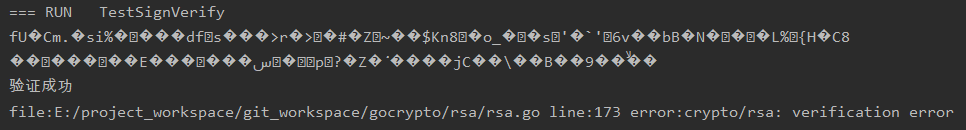# 参考

《现代密码学教程 谷利泽 杨义先等》

Go标准库-crypto/rsa

Go标准库-crypto/sha512

更多内容查看：网络安全-自学笔记

更多Go相关内容：Go-Golang学习总结笔记

有问题请下方评论，转载请注明出处，并附有原文链接，谢谢！如有侵权，请及时联系。如果您感觉有所收获，自愿打赏，可选择支付宝18833895206（小于），您的支持是我不断更新的动力。

更多相关内容
• iText数字签名官方文档参考代码 iText数字签名官方文档参考代码
• 真正完整独立运行的数字签名和密钥交换C源代码，并有教学用的课件(PDF文件), 可以到http://zhou63.ahut.edu.cn/更新。
• (纯算法，没有调用库)python实现RSA数字签名代码、ppt、文档
• 信息安全 数字签名，用的是公钥密码体制RSA算法，希望对大家有帮助数字签名
• RSA数字签名实现，采用C语言编程，代码部分粗糙，望有大佬指正，THANK YOU！
• 实现数字签名算法以及验证数字签名算法的正确性。本程序经本人验证
• 可强制签名已过期数字签名，这是成品exe，若容查杀没毒
• ## RSA数字签名源代码

热门讨论 2010-01-29 22:13:18
C# RSA数字签名代码,一般，我们可以用RSA算法生成一对密钥，公钥发放给外部客户，私钥自己保管；有以下一些应用场景： 【公钥加密、私钥解密】或者【私钥加密、公钥验证】RSA数字签名源代码
• DSA 数字签名 本程序实现DSA数字签名 是学习和研究密码学的良好资源 DSA 数字签名 本程序实现DSA数字签名 是学习和研究密码学的良好资源
• Windows 7操作系统设备驱动安装正确，但是设备管理器中出现黄色感叹号，提示信息“无法验证此设备所需驱动程序的数字签名。最近的硬件或软件更改安装的文件可能为正确签名或已损坏，或者可能来自位置来源的恶意软件...
• A会将信息用A的密码进行加密，然后将加密后的字符串和原文一起发给B，然后B用B的密码进行解密，然后判断解密后的字符串和A发过来的原文比对是否一致，关键问题在于， A和密码和B的密码不一样，这个才是数字签名的...
• 利用python实现RSA数字签名（纯算法实现），用到的hash256得到消息摘要，欧几里得，扩展欧几里得算法

# python实现RSA数字签名（纯算法实现）

## 一:什么是数字签名

数字签名是只有信息的发送者才能产生的别人无法伪造的一段数字串，这段数字串同时也是对信息的发送者发送信息真实性的一个有效证明。它是一种类似写在纸上的普通的物理签名，但是在使用了公钥加密领域的技术来实现的，用于鉴别数字信息的方法。一套数字签名通常定义两种互补的运算，一个用于签名，另一个用于验证。数字签名是非对称密钥加密技术与数字摘要技术的应用。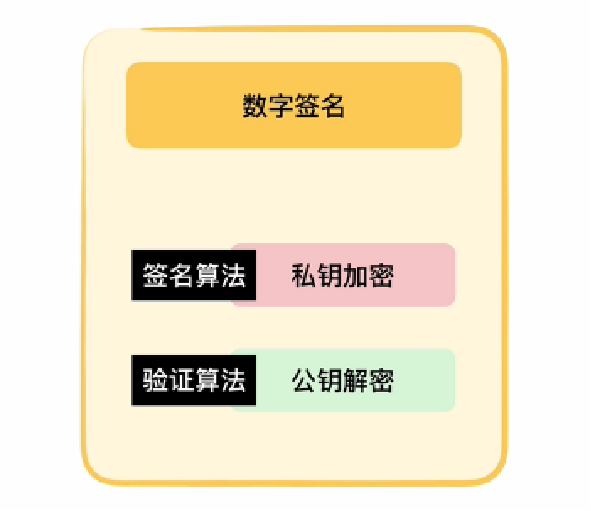## 二：数字签名的原理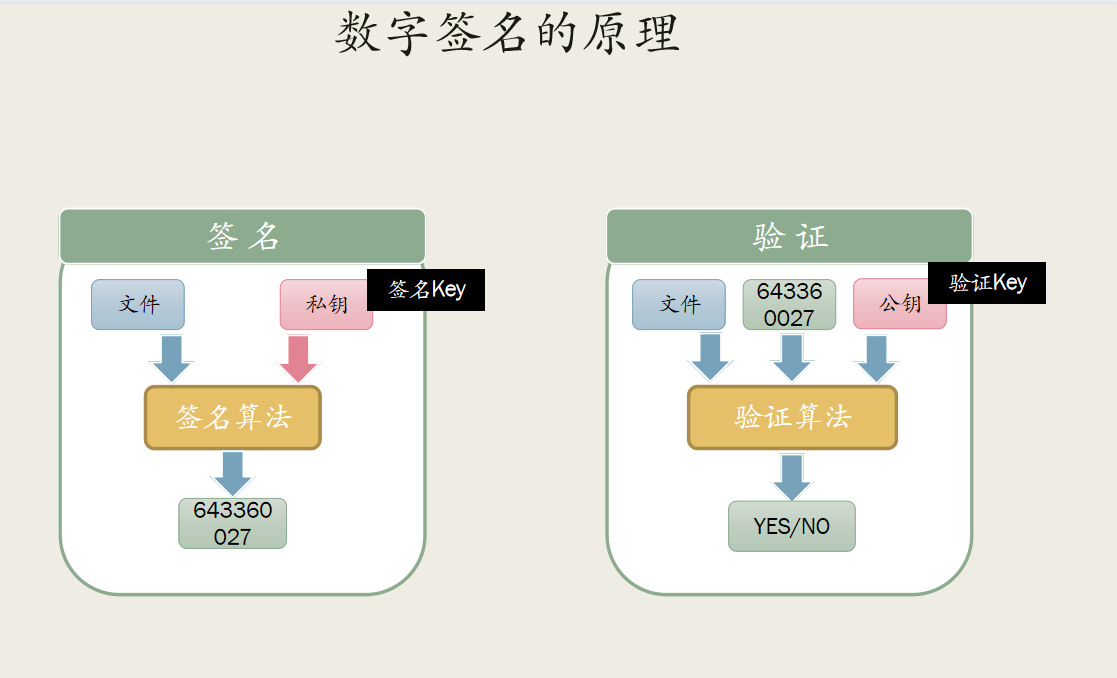发送方将先生成一对公私钥，将公钥放到网络上，发送方利用私钥将文件或者消息进行签名。接收方得到发送方的文件或者消息、公钥以及生成的签名；首先利用公钥将得到签名生成消息摘要，在对比文件或者消息的消息摘要，如果匹配，则签名认证成功。

## 三：数字签名的作用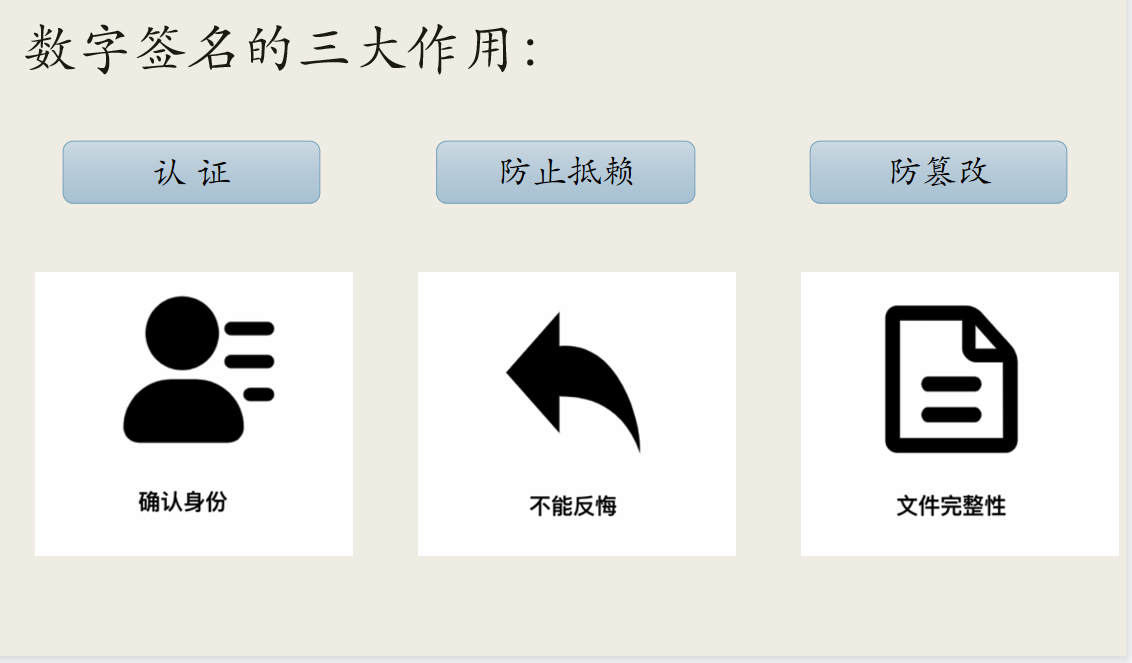## 四：实现数字签名的算法

分为三种：RSA、DSA、ECDSA

## 五：具备前提知识RSA算法

RSA加密过程：

步骤说明描述
1选择一对不相等且足够大的质数p，q
2计算p，q的乘积n=p*q
3计算n的欧拉函数φ(n)=(p-1)*(q-1)
4选一个与φ(n)互质的整数e1<e<φ(n)
5计算出e对于φ(n)的模反元素dde mod φ(n)=1
6公钥KU=(e，n)
7私钥KR=(d，n)

相关概念：

质数：质数又称素数。一个大于1的自然数，除了1和它自身外，不能被其他自然数整除的数叫做质数；否则称为合数（规定1既不是质数也不是合数）。

欧拉函数：在数论，对正整数n，欧拉函数是小于n的正整数中与n互质的数的数目

例如：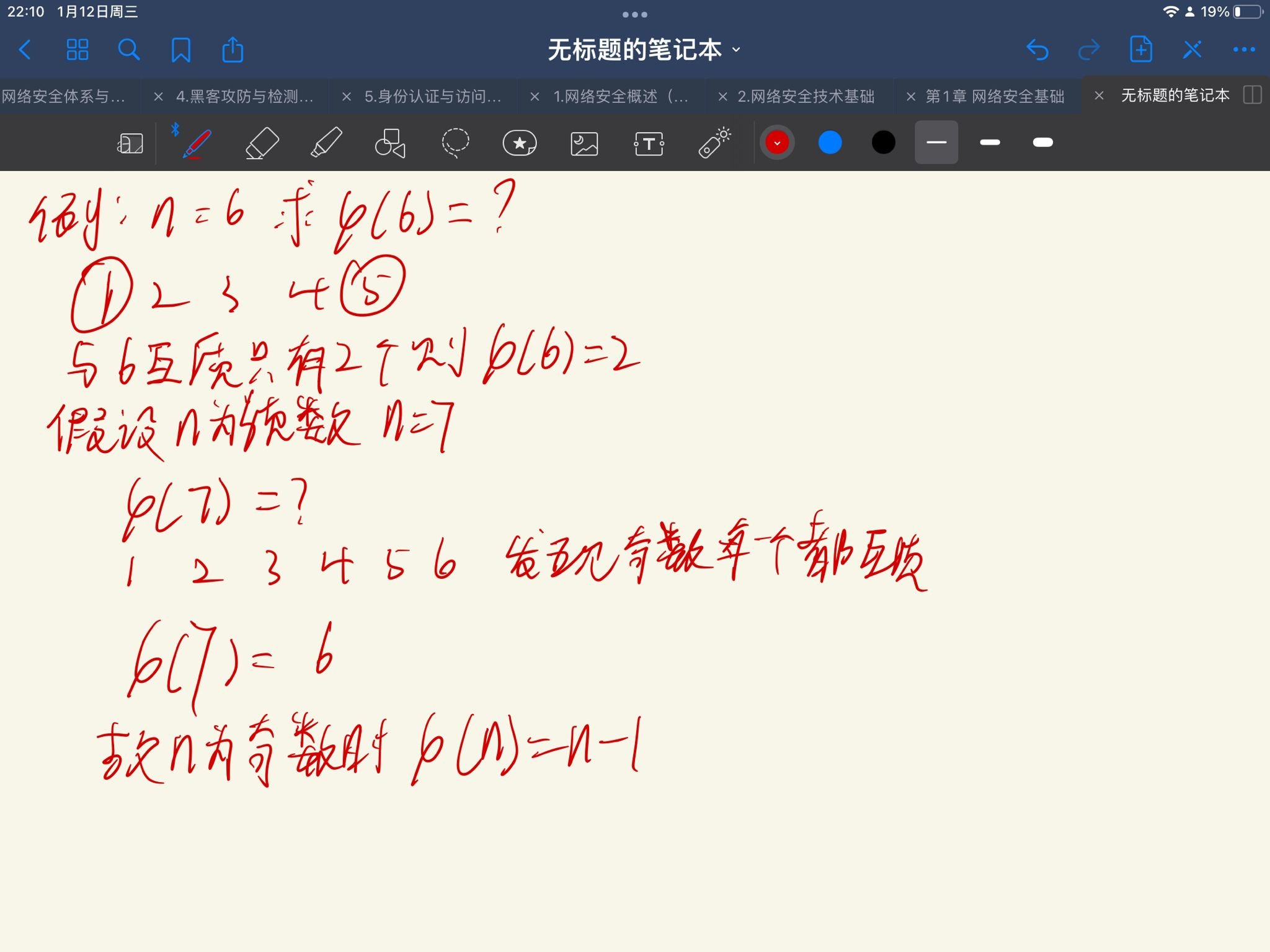• 如果n可以分解成2个质数的整数之积，那么n的欧拉函数等于这两个因子的欧拉函数之积。
• φ(n)= φ(pxq)=(p-1)*(q-1)

互质：公约数只有1的两个整数，叫做互质整数

## 六：消息摘要的前提知识

消息摘要算法的特点：

消息摘要是把任意长度的输入揉和而产生长度固定的伪随机输出的算法。消息摘要的主要特点有：

①无论输入的消息有多长，计算出来的消息摘要的长度总是固定的。例如应用MD5算法摘要的消息有128个比特位，用SHA-1算法摘要的消息最终有160比特位的输出，SHA-1的变体可以产生192比特位和256比特位的消息摘要。一般认为，摘要的最终输出越长，该摘要算法就越安全。

②消息摘要看起来是“随机的”。这些比特看上去是胡乱的杂凑在一起的。可以用大量的输入来检验其输出是否相同，一般，不同的输入会有不同的输出，而且输出的摘要消息可以通过随机性检验。但是，一个摘要并不是真正随机的，因为用相同的算法对相同的消息求两次摘要，其结果必然相同；而若是真正随机的，则无论如何都是无法重现的。因此消息摘要是“伪随机的”。

③一般地，只要输入的消息不同，对其进行摘要以后产生的摘要消息也必不相同；但相同的输入必会产生相同的输出。这正是好的消息摘要算法所具有的性质：输入改变了，输出也就改变了；两条相似的消息的摘要确不相近，甚至会大相径庭。

④消息摘要函数是无陷门的单向函数，即只能进行正向的信息摘要，而无法从摘要中恢复出任何的消息，甚至根本就找不到任何与原信息相关的信息。当然，可以采用强力攻击的方法，即尝试每一个可能的信息，计算其摘要，看看是否与已有的摘要相同，如果这样做，最终肯定会恢复出摘要的消息。但实际上，要得到的信息可能是无穷个消息之一，所以这种强力攻击几乎是无效的。

⑤好的摘要算法，没有人能从中找到“碰撞”，虽然“碰撞”是肯定存在的。即对于给定的一个摘要，不可能找到一条信息使其摘要正好是给定的。或者说，无法找到两条消息，使它们的摘要相同。

## 七：python实现消息摘要

该处我采用的是hash256: SHA256算法使用的哈希值长度是256位

python代码：

def Sha256sum(message: bytes) -> bytes:
# 定义常量
# 前8个素数2..19的平方根的小数部分的前32位
h0 = 0x6a09e667
h1 = 0xbb67ae85
h2 = 0x3c6ef372
h3 = 0xa54ff53a
h4 = 0x510e527f
h5 = 0x9b05688c
h6 = 0x1f83d9ab
h7 = 0x5be0cd19

# 定义常数K 64
# 前64个素数2..311的立方根的小数部分的前32位
K = (0x428a2f98, 0x71374491, 0xb5c0fbcf, 0xe9b5dba5, 0x3956c25b, 0x59f111f1,
0x923f82a4, 0xab1c5ed5, 0xd807aa98, 0x12835b01, 0x243185be, 0x550c7dc3,
0x72be5d74, 0x80deb1fe, 0x9bdc06a7, 0xc19bf174, 0xe49b69c1, 0xefbe4786,
0x0fc19dc6, 0x240ca1cc, 0x2de92c6f, 0x4a7484aa, 0x5cb0a9dc, 0x76f988da,
0x983e5152, 0xa831c66d, 0xb00327c8, 0xbf597fc7, 0xc6e00bf3, 0xd5a79147,
0x06ca6351, 0x14292967, 0x27b70a85, 0x2e1b2138, 0x4d2c6dfc, 0x53380d13,
0x650a7354, 0x766a0abb, 0x81c2c92e, 0x92722c85, 0xa2bfe8a1, 0xa81a664b,
0xc24b8b70, 0xc76c51a3, 0xd192e819, 0xd6990624, 0xf40e3585, 0x106aa070,
0x19a4c116, 0x1e376c08, 0x2748774c, 0x34b0bcb5, 0x391c0cb3, 0x4ed8aa4a,
0x5b9cca4f, 0x682e6ff3, 0x748f82ee, 0x78a5636f, 0x84c87814, 0x8cc70208,
0x90befffa, 0xa4506ceb, 0xbef9a3f7, 0xc67178f2)

# R为循环右移，
# 右移之后可能会超过32位，所以要和0xffffffff做与运算，确保结果为32位。
R = lambda x, n: ((x >> n) | (x << (32 - n))) & 0xffffffff
# 大端  0x12,0x34,0x56,0x78 -> 0x12345678
W = lambda i1, i2, i3, i4: (i1 << 24) | (i2 << 16) | (i3 << 8) | i4

# 对每一个输入先添加一个'0x80'，即'10000000', 即128
ascii_list = list(map(lambda x: x, message))
msg_length = len(ascii_list) * 8
ascii_list.append(128)

# 补充0
while (len(ascii_list) * 8 + 64) % 512 != 0:
ascii_list.append(0)

# 最后64为存放消息长度，以大端数存放。
# 例如，消息为'a'，则长度为'0x0000000000000008'
for i in range(8):
ascii_list.append(msg_length >> (8 * (7 - i)) & 0xff)

# print(ascii_list)
# print(len(ascii_list)//64)
for i in range(len(ascii_list) // 64):  # 64*8=512bits
# print(ascii_list[i*64:(i+1)*64])
# 每个512bits的块进行循环
w = []
# 将512bits扩展到64*32bits=2048bits存入32位无符号数数组
for j in range(16):
s = i * 64 + j * 4
w.append(W(ascii_list[s], ascii_list[s + 1], ascii_list[s + 2], ascii_list[s + 3]))
for j in range(16, 64):
s0 = (R(w[j - 15], 7)) ^ (R(w[j - 15], 18)) ^ (w[j - 15] >> 3)
s1 = (R(w[j - 2], 17)) ^ (R(w[j - 2], 19)) ^ (w[j - 2] >> 10)
w.append((w[j - 16] + s0 + w[j - 7] + s1) & 0xffffffff)
# print(hex(s0)+':'+hex(s1)+':' + hex(R(w[j - 2], 17)))
# 初始化
a, b, c, d, e, f, g, h = h0, h1, h2, h3, h4, h5, h6, h7
# for j in w:
#    print(hex(j)[2:])
for j in range(64):
s0 = R(a, 2) ^ R(a, 13) ^ R(a, 22)
maj = (a & b) ^ (a & c) ^ (b & c)
t2 = s0 + maj
s1 = R(e, 6) ^ R(e, 11) ^ R(e, 25)
ch = (e & f) ^ ((~e) & g)
t1 = h + s1 + ch + K[j] + w[j]
h = g & 0xffffffff
g = f & 0xffffffff
f = e & 0xffffffff
e = (d + t1) & 0xffffffff
d = c & 0xffffffff
c = b & 0xffffffff
b = a & 0xffffffff
a = (t1 + t2) & 0xffffffff

h0 = (h0 + a) & 0xffffffff
h1 = (h1 + b) & 0xffffffff
h2 = (h2 + c) & 0xffffffff
h3 = (h3 + d) & 0xffffffff
h4 = (h4 + e) & 0xffffffff
h5 = (h5 + f) & 0xffffffff
h6 = (h6 + g) & 0xffffffff
h7 = (h7 + h) & 0xffffffff

digest = (h0 << 224) | (h1 << 192) | (h2 << 160) | (h3 << 128)
digest |= (h4 << 96) | (h5 << 64) | (h6 << 32) | h7
# print(hex(digest)[2:])  # .rjust(32, '0'))
return hex(digest)[2:]  # .rjust(32, '0')
if __name__=="__main__":
aa='你好，中国'.encode('utf-8')
print(Sha256sum(aa))
print(len(Sha256sum(aa)))


消息摘要运行效果：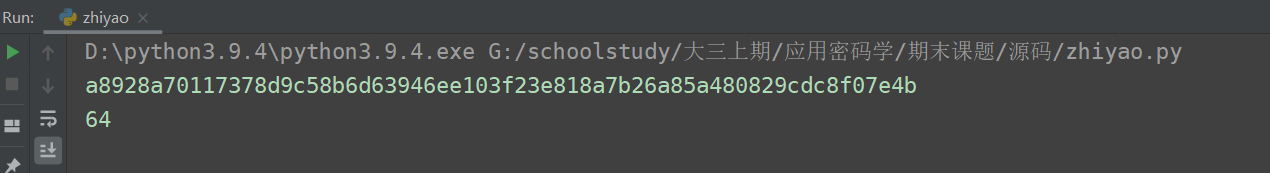## 八：RSA公私钥生成

生成公钥与私钥：此步骤按照第五步

明文 M 加密 Me mod n= C
密文 C 解密 Cd mod n=M

#D、E和N的密钥生成
def generatePublicAndSecretKeys(size = 5):
p, q = randPrime(size), randPrime(size) #生成一对不相等且足够大的质数
N = p * q #计算p、q的乘积
f = (p - 1) * (q - 1) #计算n的欧拉函数
e = randGcd1(f) #选出一个与f互质的整数e
d = liyuan(e, f)#计算出e对于f的模反元素d  de mod f =1
keys = {'d' :  d, 'e' : e, 'n' : N} #得出公钥与私钥
return keys


1、选择一对不相等且足够大的质数 p，q

#确定素数
def isPrime(num):
if (num < 2):
return False
else:
i = 2
flag=True
while i < num:
# 如果num能被i整除，说明num不是质数
if num % i == 0:
# 只要num不是质数，将flag的值修改为 False
flag = False
i += 1
return  flag
#大质数生成
def randPrime(n):
rangeStart = 10 ** (n-1) #10**4
rangeEnd = (10 ** n) - 1  #10**5-1
while True:
num = random.randint(rangeStart, rangeEnd)  #返回rangestart到rangeend任意一个数
if isPrime(num): #判断是否是质数，如果是则生成
return num


2、寻找与f互质的整数e,利用欧几里算法，如果值等于1·，那么这两个数互质

#寻找与f互质整数e
def randGcd1(b):
rangeStart = 2
rangeEnd = b - 1
while True:
num = random.randint(rangeStart, rangeEnd)
if oujilide(num, b) == 1: #利用欧几里算法，如果值等于1，那么这个两个数互质
return num
#欧几里得算法
def oujilide(a,b):
if a > b:
x = a
y = b
else:
x = b
y = a
while True:
if y == 0:
return x
else:
r = x % y
x = y
y = r


3、使用扩展欧几里得算法，计算出e对于φ(n)的模反元素d。

#扩展欧几里得算法，求逆元
def liyuan(a, n):
x1, x2, x3 = 1, 0, n
y1, y2, y3 = 0, 1, a
while y3 != 1 and y3 != 0 and y3 > 0:
Q = math.floor(x3 / y3)
t1, t2, t3 = x1 - Q * y1, x2 - Q * y2, x3 - Q * y3
x1, x2, x3 = y1, y2, y3
y1, y2, y3 = t1, t2, t3
if y3 == 0:
return 0
if y3 == 1:
if y2 >0:
return y2
else:
return n+y2


4、SHA256算法得到消息摘要，并哈希值转为整型
将消息摘要进行数字签名与验证

#SHA256算法得到消息摘要
def hashing(M, size = 5):
aa=zy.Sha256sum(M) #得到哈希值
cc=int(aa, 16) % 10 ** (size * 2 - 2)#将哈希值转化为整型
return cc
#将消息摘要进行签名
def signMessage(M, d, N):
s = power(M, d, N) #使用私钥签名 hashM**d mod N 得到签名内容
return s
#将得到
def verifySign(s, e,n):
w = power(s, e, n)
return w



## 九：实现数字签名的整体实现代码

摘要：zaiyao.py

def Sha256sum(message: bytes) -> bytes:
# 定义常量
# 前8个素数2..19的平方根的小数部分的前32位
h0 = 0x6a09e667
h1 = 0xbb67ae85
h2 = 0x3c6ef372
h3 = 0xa54ff53a
h4 = 0x510e527f
h5 = 0x9b05688c
h6 = 0x1f83d9ab
h7 = 0x5be0cd19

# 定义常数K 64
# 前64个素数2..311的立方根的小数部分的前32位
K = (0x428a2f98, 0x71374491, 0xb5c0fbcf, 0xe9b5dba5, 0x3956c25b, 0x59f111f1,
0x923f82a4, 0xab1c5ed5, 0xd807aa98, 0x12835b01, 0x243185be, 0x550c7dc3,
0x72be5d74, 0x80deb1fe, 0x9bdc06a7, 0xc19bf174, 0xe49b69c1, 0xefbe4786,
0x0fc19dc6, 0x240ca1cc, 0x2de92c6f, 0x4a7484aa, 0x5cb0a9dc, 0x76f988da,
0x983e5152, 0xa831c66d, 0xb00327c8, 0xbf597fc7, 0xc6e00bf3, 0xd5a79147,
0x06ca6351, 0x14292967, 0x27b70a85, 0x2e1b2138, 0x4d2c6dfc, 0x53380d13,
0x650a7354, 0x766a0abb, 0x81c2c92e, 0x92722c85, 0xa2bfe8a1, 0xa81a664b,
0xc24b8b70, 0xc76c51a3, 0xd192e819, 0xd6990624, 0xf40e3585, 0x106aa070,
0x19a4c116, 0x1e376c08, 0x2748774c, 0x34b0bcb5, 0x391c0cb3, 0x4ed8aa4a,
0x5b9cca4f, 0x682e6ff3, 0x748f82ee, 0x78a5636f, 0x84c87814, 0x8cc70208,
0x90befffa, 0xa4506ceb, 0xbef9a3f7, 0xc67178f2)

# R为循环右移，
# 右移之后可能会超过32位，所以要和0xffffffff做与运算，确保结果为32位。
R = lambda x, n: ((x >> n) | (x << (32 - n))) & 0xffffffff
# 大端  0x12,0x34,0x56,0x78 -> 0x12345678
W = lambda i1, i2, i3, i4: (i1 << 24) | (i2 << 16) | (i3 << 8) | i4

# 对每一个输入先添加一个'0x80'，即'10000000', 即128
ascii_list = list(map(lambda x: x, message))
msg_length = len(ascii_list) * 8
ascii_list.append(128)

# 补充0
while (len(ascii_list) * 8 + 64) % 512 != 0:
ascii_list.append(0)

# 最后64为存放消息长度，以大端数存放。
# 例如，消息为'a'，则长度为'0x0000000000000008'
for i in range(8):
ascii_list.append(msg_length >> (8 * (7 - i)) & 0xff)

# print(ascii_list)
# print(len(ascii_list)//64)
for i in range(len(ascii_list) // 64):  # 64*8=512bits
# print(ascii_list[i*64:(i+1)*64])
# 每个512bits的块进行循环
w = []
# 将512bits扩展到64*32bits=2048bits存入32位无符号数数组
for j in range(16):
s = i * 64 + j * 4
w.append(W(ascii_list[s], ascii_list[s + 1], ascii_list[s + 2], ascii_list[s + 3]))
for j in range(16, 64):
s0 = (R(w[j - 15], 7)) ^ (R(w[j - 15], 18)) ^ (w[j - 15] >> 3)
s1 = (R(w[j - 2], 17)) ^ (R(w[j - 2], 19)) ^ (w[j - 2] >> 10)
w.append((w[j - 16] + s0 + w[j - 7] + s1) & 0xffffffff)
# print(hex(s0)+':'+hex(s1)+':' + hex(R(w[j - 2], 17)))
# 初始化
a, b, c, d, e, f, g, h = h0, h1, h2, h3, h4, h5, h6, h7
# for j in w:
#    print(hex(j)[2:])
for j in range(64):
s0 = R(a, 2) ^ R(a, 13) ^ R(a, 22)
maj = (a & b) ^ (a & c) ^ (b & c)
t2 = s0 + maj
s1 = R(e, 6) ^ R(e, 11) ^ R(e, 25)
ch = (e & f) ^ ((~e) & g)
t1 = h + s1 + ch + K[j] + w[j]
h = g & 0xffffffff
g = f & 0xffffffff
f = e & 0xffffffff
e = (d + t1) & 0xffffffff
d = c & 0xffffffff
c = b & 0xffffffff
b = a & 0xffffffff
a = (t1 + t2) & 0xffffffff

h0 = (h0 + a) & 0xffffffff
h1 = (h1 + b) & 0xffffffff
h2 = (h2 + c) & 0xffffffff
h3 = (h3 + d) & 0xffffffff
h4 = (h4 + e) & 0xffffffff
h5 = (h5 + f) & 0xffffffff
h6 = (h6 + g) & 0xffffffff
h7 = (h7 + h) & 0xffffffff

digest = (h0 << 224) | (h1 << 192) | (h2 << 160) | (h3 << 128)
digest |= (h4 << 96) | (h5 << 64) | (h6 << 32) | h7
# print(hex(digest)[2:])  # .rjust(32, '0'))
return hex(digest)[2:]  # .rjust(32, '0')
if __name__=="__main__":
aa='你好，中国'.encode('utf-8')
print(Sha256sum(aa))
print(len(Sha256sum(aa)))


RSA公私钥生成：shuziqianming.py

import random
import math
import zhaiyao as zy
#确定素数
def isPrime(num):
if (num < 2):
return False
else:
i = 2
flag=True
while i < num:
# 如果num能被i整除，说明num不是质数
if num % i == 0:
# 只要num不是质数，将flag的值修改为 False
flag = False
i += 1
return  flag
#大质数生成
def randPrime(n):
rangeStart = 10 ** (n-1) #10**4
rangeEnd = (10 ** n) - 1  #10**5-1
while True:
num = random.randint(rangeStart, rangeEnd)  #返回rangestart到rangeend任意一个数
if isPrime(num): #判断是否是质数，如果是则生成
return num
#扩展欧几里得算法，求逆元
def liyuan(a, n):
x1, x2, x3 = 1, 0, n
y1, y2, y3 = 0, 1, a
while y3 != 1 and y3 != 0 and y3 > 0:
Q = math.floor(x3 / y3)
t1, t2, t3 = x1 - Q * y1, x2 - Q * y2, x3 - Q * y3
x1, x2, x3 = y1, y2, y3
y1, y2, y3 = t1, t2, t3
if y3 == 0:
return 0
if y3 == 1:
if y2 >0:
return y2
else:
return n+y2

#寻找与f互质整数e
def randGcd1(b):
rangeStart = 2
rangeEnd = b - 1
while True:
num = random.randint(rangeStart, rangeEnd)
if oujilide(num, b) == 1: #利用欧几里算法，如果值等于1，那么这个两个数互质
return num
#欧几里得算法
def oujilide(a,b):
if a > b:
x = a
y = b
else:
x = b
y = a
while True:
if y == 0:
return x
else:
r = x % y
x = y
y = r
#从数字幂快速搜索模块
def power(x, n, mod):  #x**n mod mod
if n == 0:
return 1
elif n % 2 == 0:
p = power(x, n / 2, mod)
return (p * p) % mod
else:
return (x * power(x, n - 1, mod)) % mod
#D、E和N的密钥生成
def generatePublicAndSecretKeys(size = 5):
p, q = randPrime(size), randPrime(size) #生成一对不相等且足够大的质数
N = p * q #计算p、q的乘积
f = (p - 1) * (q - 1) #计算n的欧拉函数
e = randGcd1(f) #选出一个与f互质的整数e
d = liyuan(e, f)#计算出e对于f的模反元素d  de mod f =1
keys = {'d' :  d, 'e' : e, 'n' : N} #得出公钥与私钥
return keys
#SHA256算法得到消息摘要
def hashing(M, size = 5):
aa=zy.Sha256sum(M) #得到哈希值
cc=int(aa, 16) % 10 ** (size * 2 - 2)#将哈希值转化为整型
return cc
#将消息摘要进行签名
def signMessage(M, d, N):
s = power(M, d, N) #使用私钥签名 hashM**d mod N 得到签名内容
return s
#将得到
def verifySign(s, e,n):
w = power(s, e, n)
return w



## 十：运行截图

1、对汉字的数字签名运行结果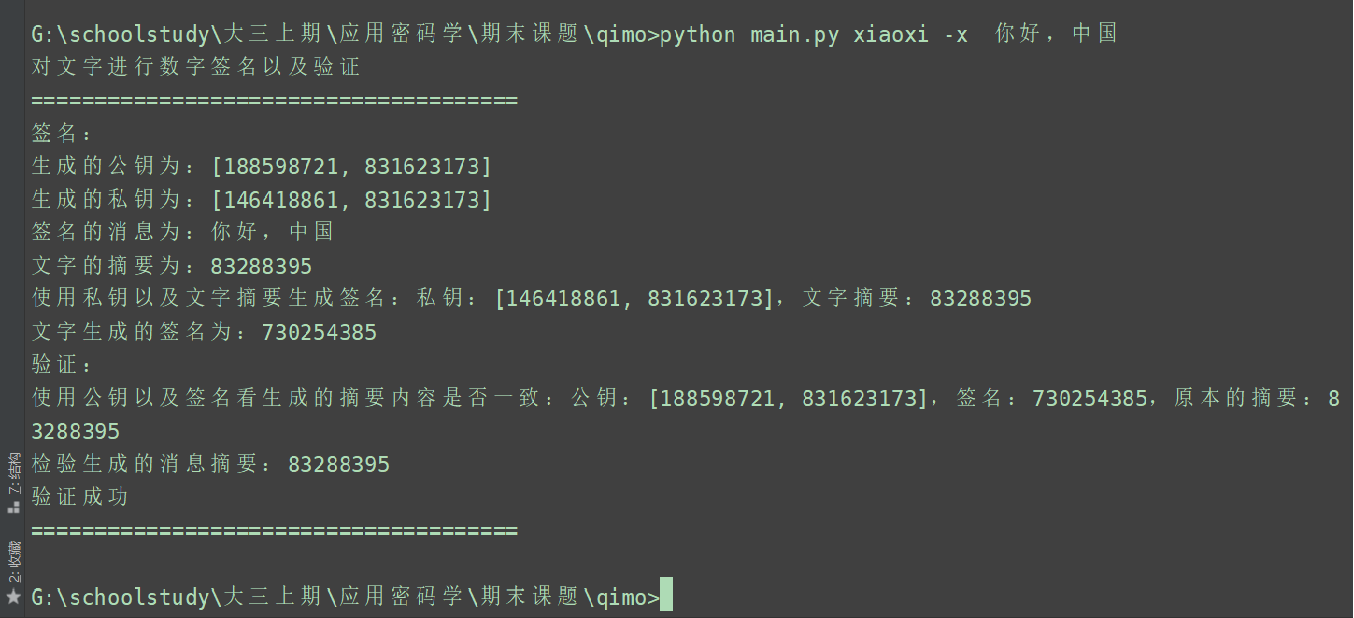2、对英文hello world 进行数字签名的运行结果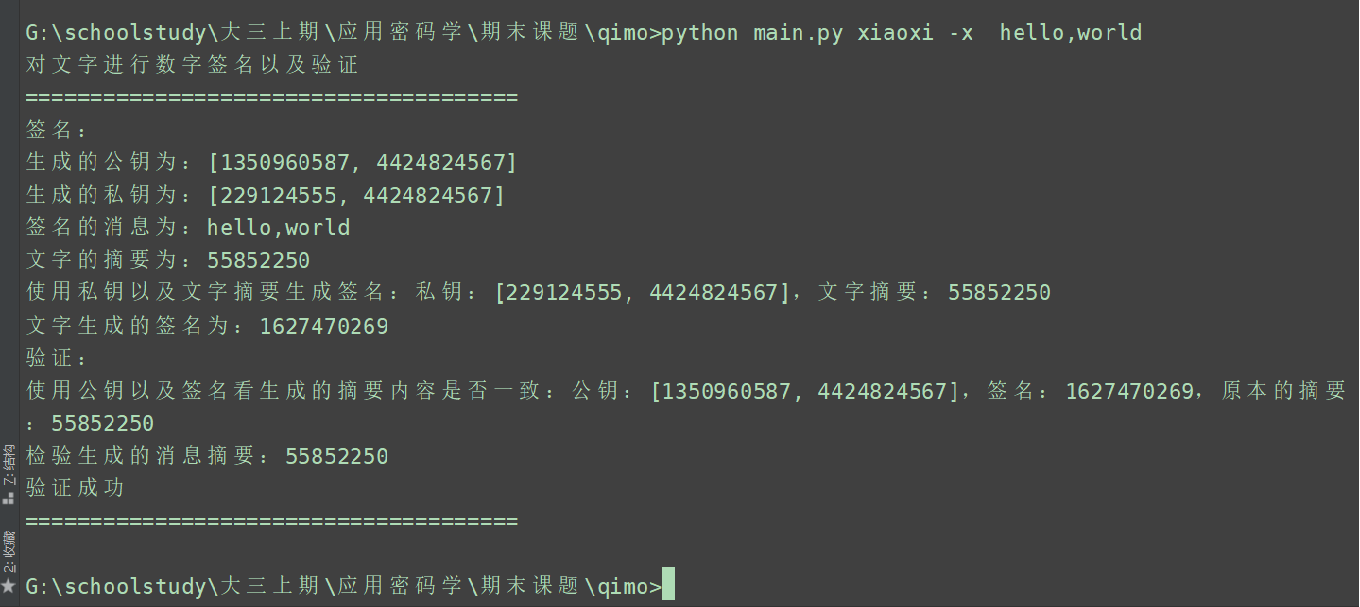3、对后缀为.png图片生成数字签名的运行结果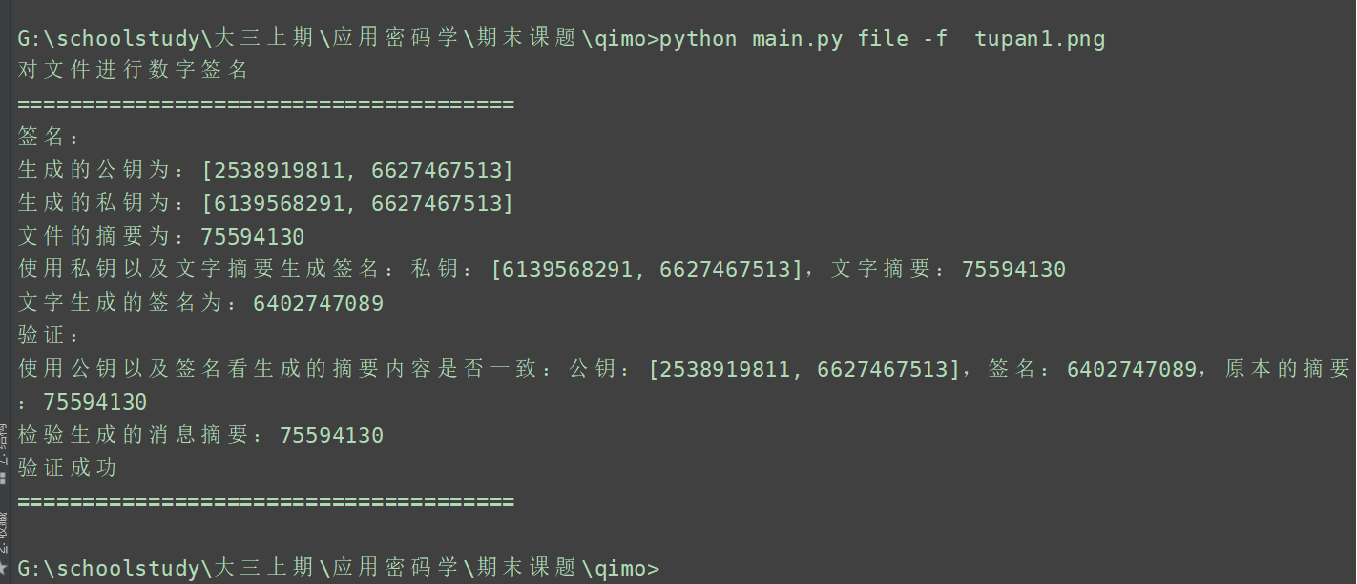4、对后缀为.doc的文档进行数字签名的运行结果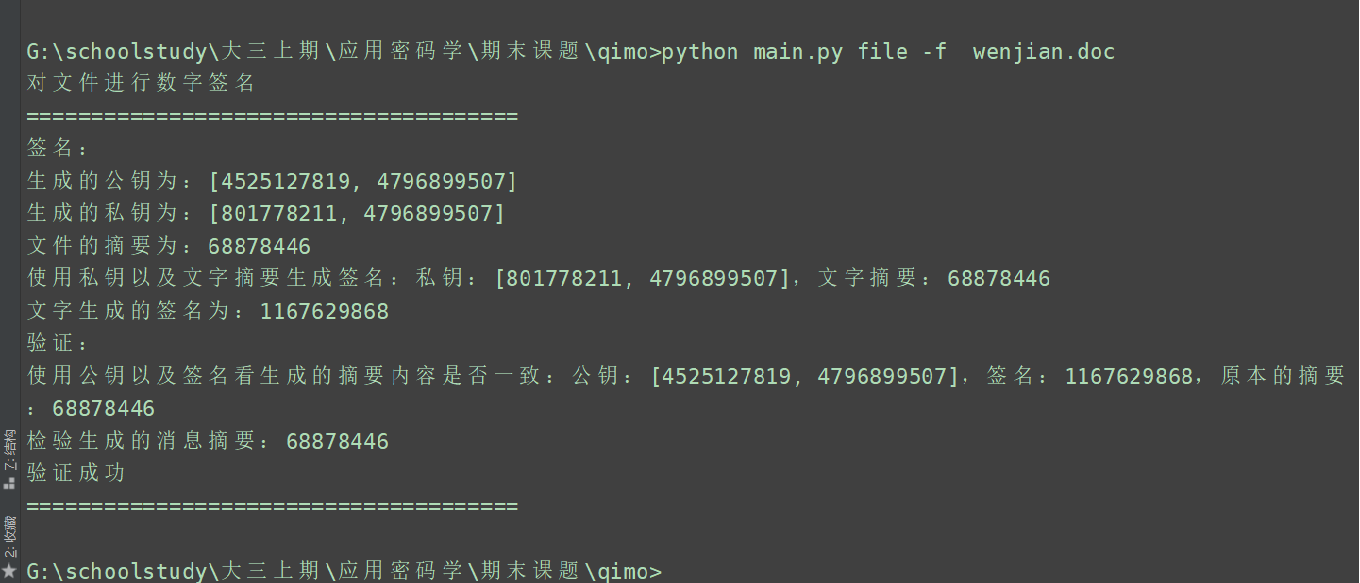## 十一：整体代码

此处没有写出主函数，如要参考，请参考github

如要参加完整代码、ppt讲解、文档：请参考(纯算法，没有调用库)python实现RSA数字签名代码、ppt、文档.rar

### 最后：如有任何问题，可以私信博主

看到博主编写文章不容易，不点赞，关注，收藏在走吗？？？展开全文python 数字签名
• 以电子形式存在于数据信息之中的，或作为其附件的或逻辑上与之有联系的数据，可用于辨别数据签署人的身份，并表明签署人对数据信息中包含的信息的认可。数字签名
• 详细的rsa数字签名代码，使用的是c++语言，可以通过编译
• 在通过精美的包装盒销售软件的时代，...但在当今的网络时代，有利的一面是软件开发商可以通过网络不受时间、地域的限制而快速发行软件，但不利的一面，则是用户无法辨认软件的真伪，根本无法确认软件代码的真实身份。
• Elgamal数字签名主要利用离散对数的特性来实现签名，具体方式如下： 生成 随机选择 签名算法 验证算法
• 在Harn广播多重数字签名方案的基础上提出了一种广播多重(t,n)门限数字签名方案，该方案系统初始化简单、安全性较好、通信成本低，对于广播多重(t,n)门限签名消息在网络上的传输和处理具有实用价值。
• 代码签名数字证书（含私钥）,数字签名是使用自己的私钥签名,C,C++源码.zip
• 故障描述：Windows 7操作系统设备驱动安装正确，但是设备管理器中出现黄色感叹号，提示信息“无法验证此设备所需驱动程序的数字签名。最近的硬件或软件更改安装的文件可能为正确签名或已损坏，或者可能来自位置来源...
• 利用rsa将输入的文本进行加密。解密有些问题，如需要可以下载我的数字签名资源
• 可强制签名已过期数字签名，这是成品exe，若容查杀没毒
• 数字签名，经常也被称做电子签名，是用来建立电子传送的报文或电子文档的可靠性的。核查数字签名便有可能断定报文或文档是否被改动过。 　签名具有的特性是它只能由单独的某个人正确产生，但它可以——或至少由任何...
• 解决win7 64位驱动程序签名强制问题，即在设备管理器，设备驱动呈现黄色感叹号，提示为Windows无法验证此设备所需的驱动程序的数字签名。。。（代码 52） 网上教程：重启按F8 选择禁用驱动程序签名强制，但每次重启...
• Windows无法验证文件数字签名而无法启动，无法验证此驱动的数字签名的网络上的解决方案一般都是下面几种： 第一种： 开机时按F8键，在弹出的菜单中有 禁用驱动程序签名强制 选项，使用上下键选中之后，再按回车键......